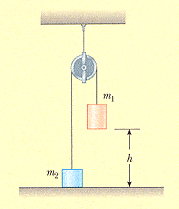# Conservation of engery of a pulley system

jwxie

## Homework Statement

Two objects, m1 = 4.50 kg and m2 = 3.00 kg, are connected by a light string passing over a light frictionless pulley as shown in the figure below. The object of mass 4.50 kg is released from rest, h = 3.00 m above the ground.(a) Using the isolated system model, determine the speed of the 3.00 kg object just as the 4.50 kg object hits the ground.
(ans: 4.43)

(b) Find the maximum height to which the 3.00 kg object rises.
(ans: 5)

## Homework Equations

$$\Delta K$$ = - [tex]\Delta Ug[/text]
mgy - mgyf = - [tex]\Delta Ug[/text]
KEi + PEi = KEf + PEf

## The Attempt at a Solution

I know I can solve problem a differently. Suppose I solve each mass separately using law of conservation of energy, I can find Vf for mass 2 separately.

Since the system is connected by the same uniform massless string, so the accerlation must the same. But I did not assume their Vf will be the same (the impact of m1 as it hits the ground, and at that instant the Vf of m2 raising to 4 meter).

What I want to know is, how do you prove that the final velocity of m1 and m2 at that same instant is the same using the isolated system model KEf + PEf = KEi + PEi ?

I have the following data on my hands

for m2, where m= m2, h = 4
_________________________
| | KE | PE |
| i | 0 | 0 |
| f | 1/2mv^2 | m2h |

for m1 where m = m1, h = 4
| | KE | PE |
| i | 1/2mv^2 | mgh |
| f | 0 | 0 |

Last edited by a moderator: